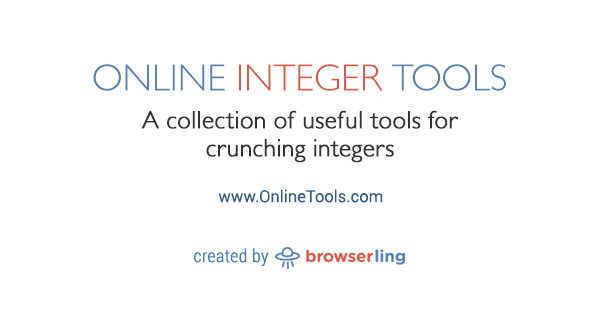At Browserling we're building a network of online tools websites. Each site focuses on one and only one tool category. Each tool does one and only one thing.

Today we're releasing the twentieth site – Online Integer Tools.

Online Integer Tools offers a collection of simple, free, and easy to use utilities for crunching integers. There are no intrusive ads, popups, or other garbage, just integer utilities that work in your browser. Load integers and instantly get the result!www.onlineINTEGERtools.com

Here's a list of all integer tools so far:

Here are the upcoming integer tools:

• Draw von Neumann Universe
• Let Zalgo Destroy Integers
• Create Integer n-Tuples
• Convert Integers to Unary Base
• Convert Unary Base to Integers
• Convert Integers to Binary Base
• Convert Binary Base to Integers
• Convert Integers to Octal Base
• Convert Octal Base to Integers
• Convert Integers to Hex Base
• Convert Hex Base to Integers
• Convert Integers to Any Base
• Convert Integers to Base64
• Convert Base64 to Integers
• Convert Integers to HTML Entities
• Convert HTML Entities to Integers
• Create Random Integers
• Create Palindromic Integers
• Check if an Integer is Symmetric
• Generate an Integer Matrix
• Rewrite an Integer as a Sum
• Rewrite an Integer as a Product
• Rotate Integers
• Shift Integer Digits
• Subtract Integers
• Multiply Integers
• AND Integers
• OR Integers
• XOR Integers
• NOT Integers
• NAND Integers
• NOR Integers
• XNOR Integers
• Find the Running Total
• Find the Running Product
• Convert an Integer to Ordinal
• Convert an Oridinal to Integer
• Calculate Integer Digit Sum
• Filter Integers
• Merge Integers
• Truncate Integers
• Right-align Integers
• Center Integers
• Take Absolute Value of Integers
• Make All Integers Negative
• Convert Fractions to Integers
• Analyze an Integer
• Find and Replace Integers
• Generate Integers from Regex
• Create an Integer Array

The first nineteen websites in the network are:

The next few sites are onlineHASHtools, onlineGIFtools, onlineBITMAPtools, onlinePDFtools, onlineBROWSERtools, onlineCRYPTOtools, onlineAUDIOtools, onlineCSStools, onlineJStools, and a dozen more.

See you next time!